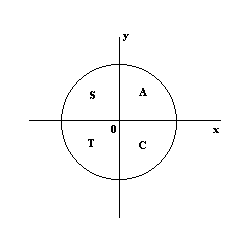# Trigonometry: The Unit Circle (Part 2)Sorry for not posting for such a long time. I have been busy with some schoolwork and i got sick.

This post is part of a series of posts on Trigonometry. To see all the posts, click on the tag #TrigonometryTutorials below. This is the post you should read before you read this.

Last time we talked about the new Definitions of the trig functions now that we know the unit circle.

$\sin \theta$ is the $y$ co-ordinate reading of the unit circle, $\cos \theta$ is the $x$ co-ordinate reading of the unit circle, and $\tan \theta$ is the $y$ divided by $x$

In Q I both $x$ and $y$ are positive, so sine, cosine, and tangent are all positive when $\theta$ is in QI.

In QII $x$ is negative but $y$ is positive, so in QII only sine is positive, and Cosine and Tangent are negative

In QIII both $x$ and $y$ are negative. Only Tangent is Positive as tangent is $y$ divided by $x$ the negatives are cancelled. Sine and Cosine are Negative in QIII

In QIV $y$ is negative but $x$ is positive, so in QIV only cosine is positive, and Sine and Tangent are negativeNote by Yan Yau Cheng
7 years, 6 months ago

This discussion board is a place to discuss our Daily Challenges and the math and science related to those challenges. Explanations are more than just a solution — they should explain the steps and thinking strategies that you used to obtain the solution. Comments should further the discussion of math and science.

When posting on Brilliant:

• Use the emojis to react to an explanation, whether you're congratulating a job well done , or just really confused .
• Ask specific questions about the challenge or the steps in somebody's explanation. Well-posed questions can add a lot to the discussion, but posting "I don't understand!" doesn't help anyone.
• Try to contribute something new to the discussion, whether it is an extension, generalization or other idea related to the challenge.

MarkdownAppears as
*italics* or _italics_ italics
**bold** or __bold__ bold
- bulleted- list
• bulleted
• list
1. numbered2. list
1. numbered
2. list
Note: you must add a full line of space before and after lists for them to show up correctly
paragraph 1paragraph 2

paragraph 1

paragraph 2

[example link](https://brilliant.org)example link
> This is a quote
This is a quote
    # I indented these lines
# 4 spaces, and now they show
# up as a code block.

print "hello world"
# I indented these lines
# 4 spaces, and now they show
# up as a code block.

print "hello world"
MathAppears as
Remember to wrap math in $$ ... $$ or $ ... $ to ensure proper formatting.
2 \times 3 $2 \times 3$
2^{34} $2^{34}$
a_{i-1} $a_{i-1}$
\frac{2}{3} $\frac{2}{3}$
\sqrt{2} $\sqrt{2}$
\sum_{i=1}^3 $\sum_{i=1}^3$
\sin \theta $\sin \theta$
\boxed{123} $\boxed{123}$

Sort by:

Keep on posting.

- 7 years, 6 months ago